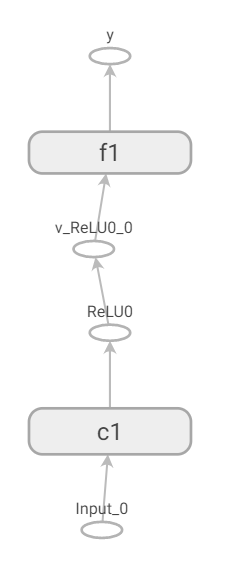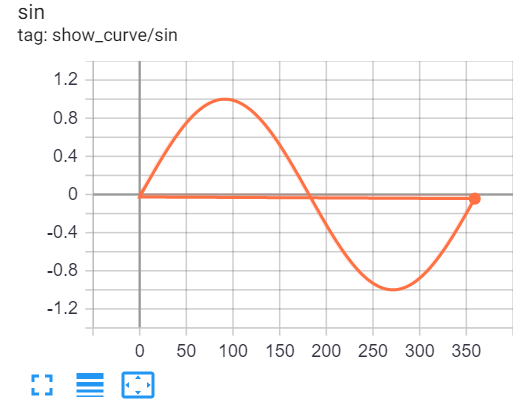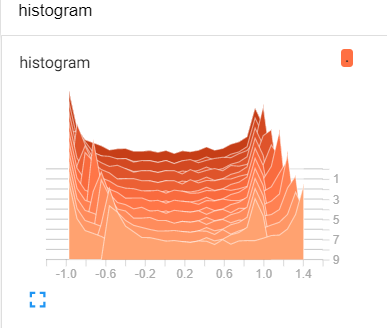# Show Graph by Tensorboard¶

## TBGraphWriter¶

class nnabla.experimental.tb_graph_writer.TBGraphWriter(log_dir='log_out', comment='', **kwargs)[source]
This class is a wrapper of tensorboardX summary writer,

which enable nn.Variable can be visualized as a graph by tensorboard.

Prerequisite:

Install tensorflow and tensorboardX, simply by the following commands:

```pip install tensorflow
pip install tensorboardX
```

Please refer to the following example to use this class:

Example:

```import numpy as np
import nnabla as nn
import nnabla.functions as F
import nnabla.parametric_functions as PF

def show_a_graph():
try:
from nnabla.experimental.tb_graph_writer import TBGraphWriter
except Exception as e:
print("please install tensorflow and tensorboardX at first.")
raise e

nn.clear_parameters()
x = nn.Variable((2, 3, 4, 4))
with nn.parameter_scope('c1'):
h = PF.convolution(x, 8, (3, 3), pad=(1, 1))
h = F.relu(PF.batch_normalization(h))
with nn.parameter_scope('f1'):
y = PF.affine(h, 10)

with TBGraphWriter(log_dir='log_out') as tb:
tb.from_variable(y, output_name="y")
```

The corresponding graph can be visualized as the following:Or, you may show scalar value or histogram of values along the increasing of iteration number as the following:

```with TBGraphWriter(log_dir='log_out') as tb:
values = []
for i in range(360):
s = np.sin(i / 180.0 * np.pi)
values.append(s)

nd_values = np.array(values)
for i in range(10):
nd_values += 0.05
```

It looks like:dThis class writes a protobuf file to `log_dir` specified folder, thus, user should launch tensorboard to specify this folder:

```tensorboard --logdir=log_out
```
Then, user may check graph in a web browser, by typing the address:

http://localhost:6006

• leaf (`nn.Variable`) – Leaf node of graph, normally, the output variable of a network